• 阅读数：158发布于2021-07-16 19:34:32

只看该作者
###### 机械臂建模，轨迹规划，避障路径规划（介绍+代码）（二） 复制本文链接

最近写的一个作业，记录分享一下，主要是对机械臂在MATLAB中的仿真，其中包括机械臂建模，机械臂轨迹规划，机械臂避障路径规划等。这部分主要记录机械臂轨迹绘制。
工作空间获取
因为要对机械臂进行轨迹绘制，所以首先对机械臂的工作空间进行大致确定，以防止规划点超出了工作空间不可达而无法进行规划。以下是仿真结果和对应程序：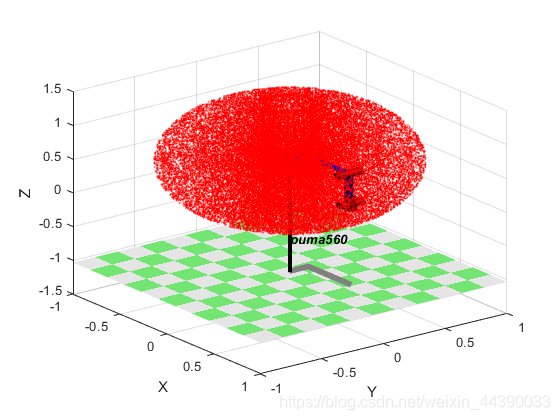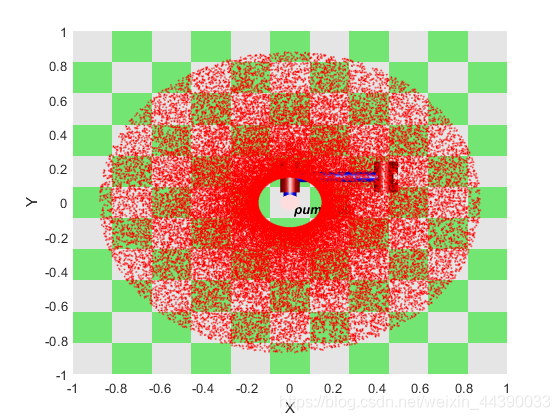``````%%
工作空间
clear;
clc;
%建立机器人模型
% theta d a alpha offset
L1=Link([0 0 0 0 0 ],'modified'); %连杆的D-H参数
L2=Link([0 0.14909 0 -pi/2 0 ],'modified');
L3=Link([0 0 0.4318 0 0 ],'modified');
L4=Link([0 0.43307 0.02032 -pi/2 0 ],'modified');
L5=Link([0 0 0 pi/2 0 ],'modified');
L6=Link([0 0 0 -pi/2 0 ],'modified');
robot=SerialLink([L1 L2 L3 L4 L5 L6],'name','puma560','base' , ...
transl(0, 0, 0.62)* trotz(0)); %连接连杆，机器人取名puma560

% 参数
%关节角限位
q1_s=-160; q1_end=160;
q2_s=-225; q2_end=45;
q3_s=-45;  q3_end=225;
q4_s=-110; q4_end=170;
q5_s=-100;  q5_end=100;
q6_s=-266;  q6_end=266;

%计算点数
num=50000;

% 求取工作空间
%设置轴关节随机分布,轴6不对工作范围产生影响，设置为0
q1_rand = q1_s + rand(num,1)*(q1_end - q1_s);
q2_rand = q2_s + rand(num,1)*(q2_end - q2_s);
q3_rand = q3_s + rand(num,1)*(q3_end - q3_s);
q4_rand = q4_s + rand(num,1)*(q4_end - q4_s);
q5_rand = q5_s + rand(num,1)*(q5_end - q5_s);
q6_rand = q6_s + rand(num,1)*(q6_end - q6_s);
q = [q1_rand q2_rand q3_rand q4_rand q5_rand q6_rand];

%正运动学计算工作空间
tic;
T_cell = cell(num,1);
[T_cell{:,1}]=robot.fkine(q).t;%正向运动学仿真函数
disp(['运行时间：',num2str(toc)]);

% 分析结果
%绘制工作空间
t1=clock;
figure('name','机械臂工作空间')
hold on
plotopt = {'noraise', 'nowrist', 'nojaxes', 'delay',0};
robot.plot([0 0 0 0 0 0], plotopt{:});
figure_x=zeros(num,1);
figure_y=zeros(num,1);
figure_z=zeros(num,1);
for cout=1:1:num
figure_x(cout,1)=T_cell{cout}(1);
figure_y(cout,1)=T_cell{cout}(2);
figure_z(cout,1)=T_cell{cout}(3);
end
plot3(figure_x,figure_y,figure_z,'r.','MarkerSize',3);
hold off
disp(['绘制工作空间运行时间：',num2str(etime(clock,t1))]);

%获取X,Y,Z空间坐标范围
Point_range=[min(figure_x) max(figure_x) min(figure_y) max(figure_y) min(figure_z) max(figure_z)];
disp(['X在空间坐标范围:',num2str(Point_range(1:2))]);
disp(['Y在空间坐标范围:',num2str(Point_range(3:4))]);
disp(['Z在空间坐标范围:',num2str(Point_range(5:6))]);
``````

轨迹规划及绘制
轨迹规划包括两种，关节空间轨迹规划和笛卡尔空间轨迹规划。
关节空间轨迹规划是对机械臂的每个关节的运行进行插值拟合，以保证机械臂的各个关节运动的连续性和稳定性。
笛卡尔空间轨迹规划常用于焊接，切割等场合，即对规定好的轨迹进行绘制，即保证机械臂末端的以确定的姿态在规定轨迹上运动。
关节空间轨迹规划
关节空间轨迹规划常用的插值方法有三次插值，五次插值等，关于五次插值具体可以看一下这个博主的文章六轴机器人轨迹规划之五次多项式插值，这里放一下效果图：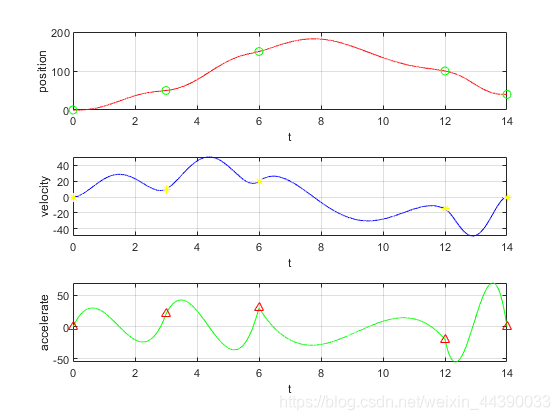可以看到，关节角度，角速度，角加速度都非常平滑。而如果要求不高则可以使用MATLAB自带的三次样条插值函数cubic，可以只对角度进行插值，代码及仿真图如下：

``````clear;
clc
ty=output1(:,1);
ty=ty';
tx=1:21;
tx1=1:0.2:21;
ty1=interp1(tx,ty,tx1,'cubic');
figure
plot(tx,ty,'o',tx1,ty1,'r');
title('三次多项式插值')
``````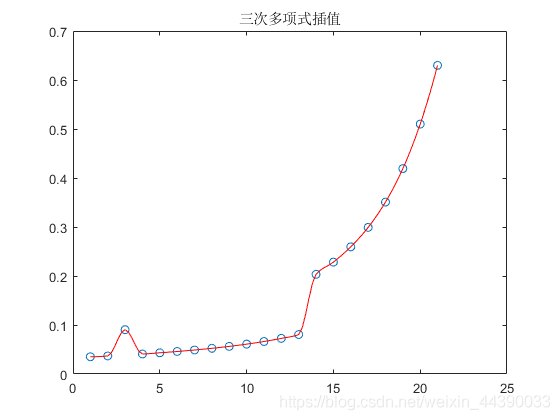笛卡尔空间轨迹规划
笛卡尔空间轨迹规划就是对机械臂末端路径进行规划，常见的有直线形，圆弧形，其他形状则由这两种变形组合。这部分较为简单，可以在相关博文中找到。
在得到直线形和圆弧形的轨迹之后，需要对机械臂进行控制，即将得到的规划轨迹点代入机械臂逆运动学中，求出每个轨迹点对应的八个逆解，求逆解的介绍在这篇博文中，机械臂建模，轨迹规划，避障路径规划（介绍+代码）（一）
再从八个逆解中按前后关节角距离最近和转角之和最小的两个原则选择出一个逆解，最后所有的轨迹点都求出了对应的逆解，再将对应的逆解代入机械臂中即可进行运动控制，仿真效果如下：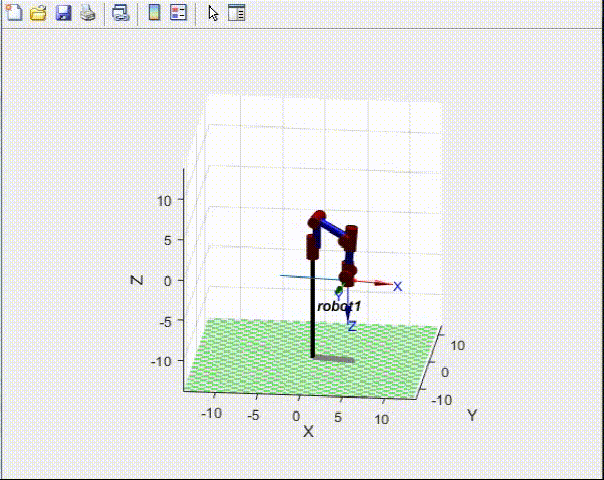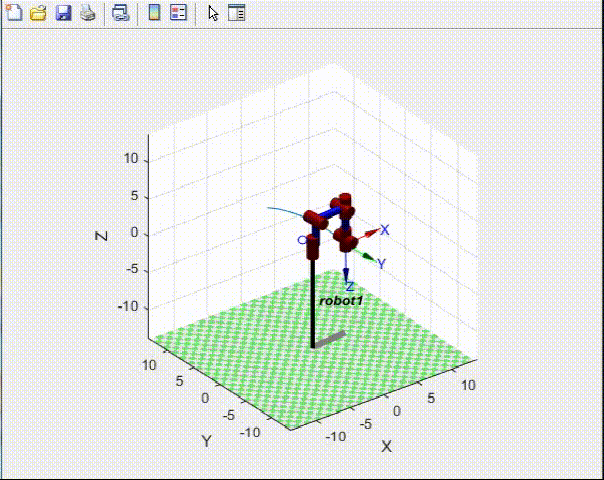举报

• 垃圾广告
• 违规内容
• 恶意灌水
• 重复发帖